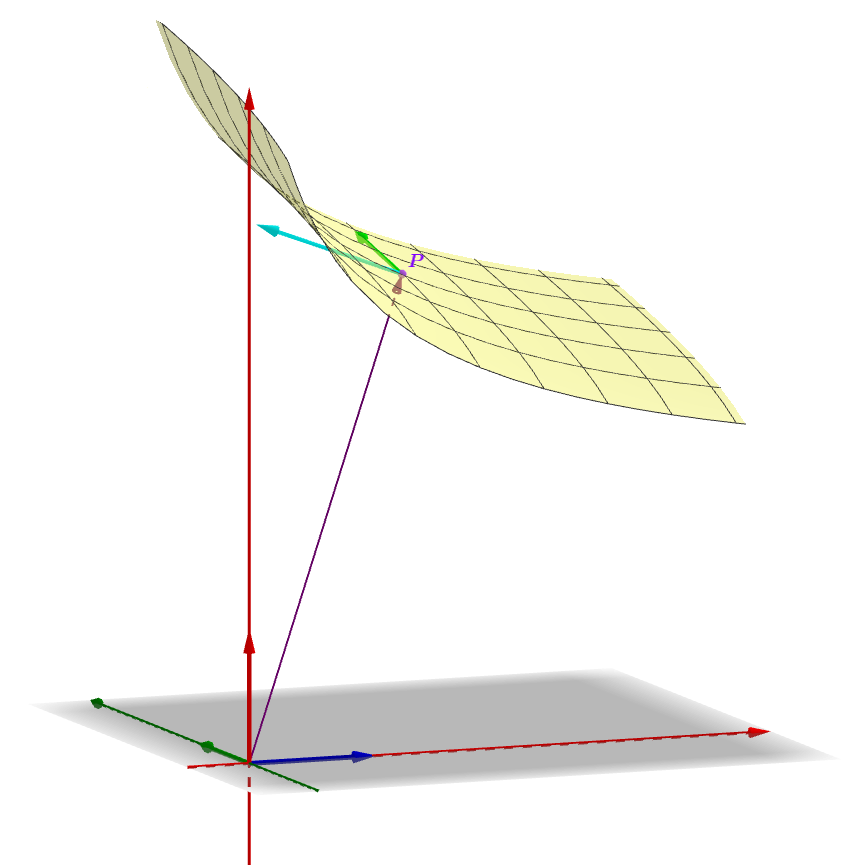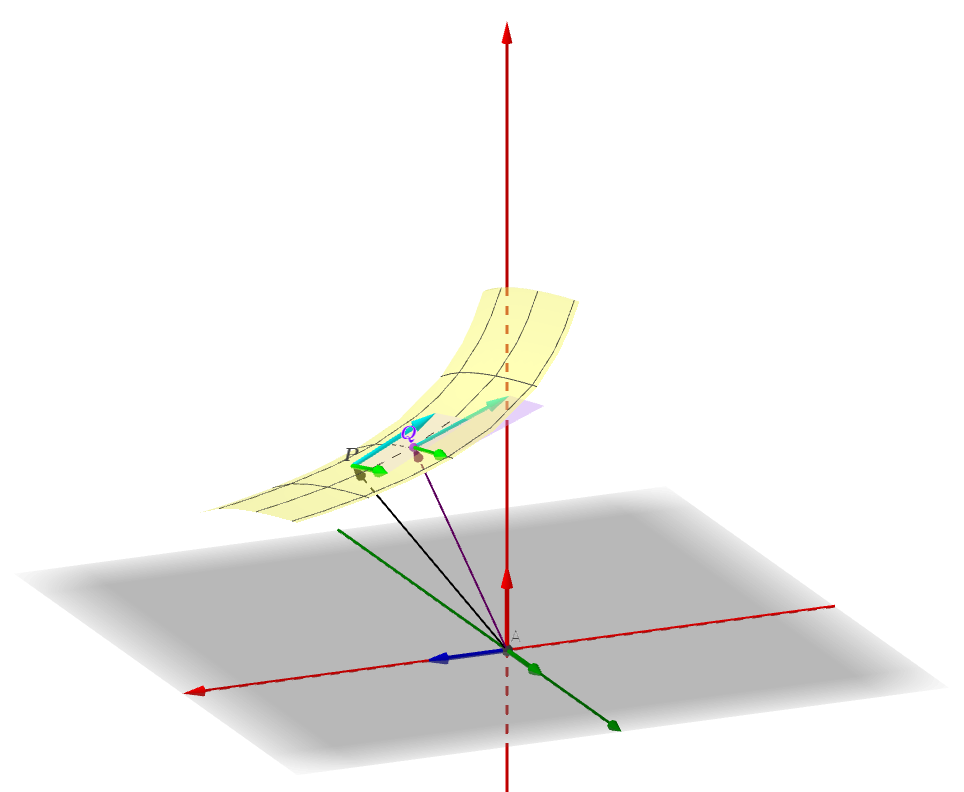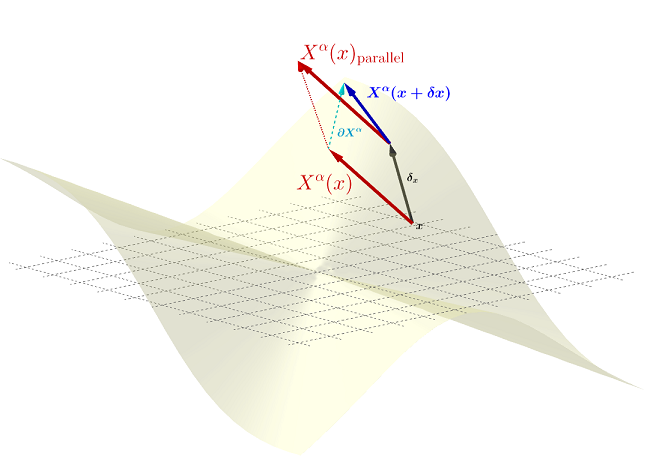### Riemannian geometry:

From this reference.

Riemannian geometry deals with intrinsic geometry on surfaces. The position vector at point P can be expressed on the basis of the extrinsic coordinates, or on the intrinsic coordinates on the manifold (the mesh of space-time). At each point of the manifold (referenced either extrinsically through the Cartesian system; or intrinsically via the curvilinear coordinate lines on the manifold), there will be a tangent vector space (and a dual). However, each point will have its own tangent vector space $$T_P(M).$$ Over the manifold, there will be a collection of vector spaces - one for each point, $$T_x(M).$$ A single vector can be selected at each point as a function of the coordinates (a function of space-time), creating a vector field:

$\underbrace{X^\alpha(x)}_{\text{components as f(space-time)}}\underbrace{\quad\partial _\alpha\quad}_{basis}\in\quad T_x(M).$The basis of the tangent space will be $$\frac{\partial}{\partial x^i}$$ or $$\partial x^i.$$

The vectors in the tangent space and in the dual of the tangent space at every point are related through the metric $$g_{\mu\nu}(x)\,dx^\mu\otimes dx^\nu,$$ which is also a function of space time. This gives us an inner product at each point. For each vector in the tangent space $$\left<\vec v,\;\right>\to \mathbb R,$$ i.e. filling in the space with any other vector will produce a real number. Hence, $<v,;>V^*,$ linking the vector space with its dual.

However, we are still stuck at any particular point in space-time. As we move differentially along the manifold, the tangent plane changes since the vector space changes with every point. So we need a connector to relate vectors across the field.The change of coordinates between two points $$P$$ and $$Q$$ separated by a differential value $$dx$$ as expressed in the extrinsic coordinate system (position vectors from the origin) could be encapsulated with the Taylor series. The new extrinsic coordinates in the extrinsic plane will be $$x+dx$$ and $$y+dy$$, and the height will be a function of these two coordinates:

$z(x+dx,y+dy)=z(x,y) + \frac{\partial z}{\partial x} dx+ \frac{\partial z}{\partial y} dy$

The distance vector $$ds$$ will be the difference between these two position vectors:

$ds=z(x+dx,y+dy)- z(x,y)=\frac{\partial z}{\partial x} dx+ \frac{\partial z}{\partial y} dy$

and the square distance (changing now to covariant notation for covectors)

$dx^2=ds \cdot ds=g_{ij}\;dx^idy^j$

with $$g_{ij}$$ constituting the metric tensor. In Cartesian coordinates the metric tensor is simply the identity matrix.

XylyXylyX labels the vector that the vector field places at $$x + \delta x,$$ i.e. the position in space-time shifted by a small increment,as $$X^\alpha(x+\delta x),$$ with a first grade Taylor expansion:

\begin{align} X^\alpha\,(x + \delta x) &= \underbrace{X^\alpha (x)}_{\text{value at }x}+\delta x^b\;\underbrace{\partial_bX^\alpha}_{\partial \text{wrt compon's } \delta x}\\[2ex] &=X^\alpha(x) + \delta x^b\;\frac{\partial X^\alpha}{\partial x^b}\\[2ex] &= X^\alpha(x) + \delta X^\alpha \end{align}

#### Covariant derivative:

Differential changes across a vector field will necessitate a way of moving across tangent spaces